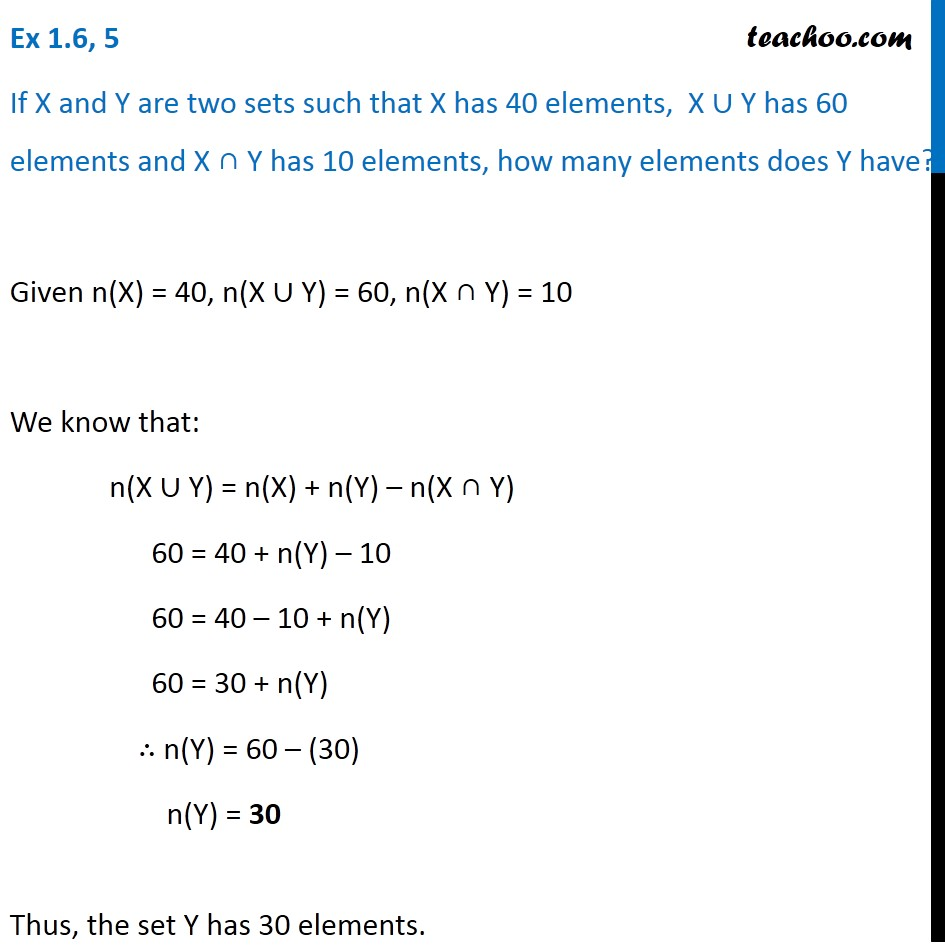Number of elements in Set Formula

Chapter 1 Class 11 Sets
Serial order wiseLearn in your speed, with individual attention - Teachoo Maths 1-on-1 Class

### Transcript

Question 5 If X and Y are two sets such that X has 40 elements, X ∪ Y has 60 elements and X ∩ Y has 10 elements, how many elements does Y have? Given n(X) = 40, n(X ∪ Y) = 60, n(X ∩ Y) = 10 We know that: n(X ∪ Y) = n(X) + n(Y) – n(X ∩ Y) 60 = 40 + n(Y) – 10 60 = 40 – 10 + n(Y) 60 = 30 + n(Y) ∴ n(Y) = 60 – (30) n(Y) = 30 Thus, the set Y has 30 elements.